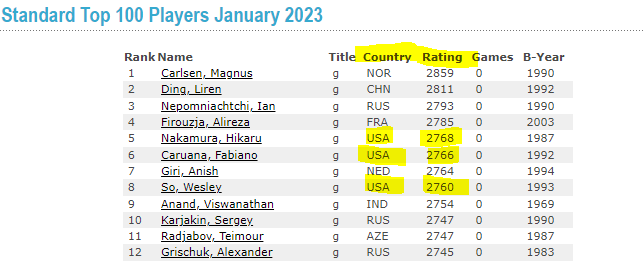# Which country has most points?

Hello,

I´m trying to complete this exercise in which I have to read this table FIDE Online. FIDE Top players - Standard Top 100 Players January 2023 into a data table and then separate a data table that contains each country once that is represented in the ranking table.

Then I should count the total ranking points for each country and add this information to the datatable I created that contains the countries.

I have tried to use for each loop to get the sum of total ranking points but can´t figure out how to get the activity to calculate correct amount of ranking points to each country.

Here is a picture of what I have been trying to do.

Thank you very much in advance!

@Comet
Looking at the Table:How do you figure the Total Ranking Points? I have highlighted USA, do you sum the three ratings? Need clarification please? Only the country and points out?

Regards

Hello,

Thank you for your reply. There are for example 13 players in total in the top 100 list from USA so I have to calculate the rating from these USA players together and insert that sum into the datatable that has the countries listed in it. This I have to do for all the countries players. There are for example 8 players from CHN and so on.

@Anil_G Can you help with this? Thank you very much if you have time to help.

@Comet

I believe you do not have any issue with scrapping the data…now to geoup by the country and get the sum for each use the following formula in assign activity…and yo save the values create a new dt with two columns one for country and one for sum using build datatable activity…sum column type can be double… and country will be string

`Newdt = (From d In ExtractedTable.AsEnumerable() Group d By k=d("Country").toString.Trim Into grp = Group Let sum = grp.sum(function(x) CDBL(x("Rating").ToString)) Let ra = New Object(){k,sum} Select r = Newdt.Rows.Add(ra)).CopyToDataTable()`

Hope this helps

Cheers

1 Like

Thank you for your quick response. This helped a lot but I still have this issue. I get this error text “Assign: Object reference not set to an instance of an object” when trying to use the formula you gave in an assign activity.

The name of the columns in the datatable seem to be “Column-1”, “Column-2” and so on. I tried to take this to account in the formula and tried to modify the datatable by removing the first row containing the “Name”, “Country” and “Rating” etc. This I did because I got an error message about that the “Rating” could not be converted from double to string.

Here is a picture of the datatable as it was when I worked on this problem.

Hi @Comet

you need to build the data table “Newdt” with “Country” as string data type and “sum” as double data type before the assign activity to avoid “Object reference not set to an instance of an object” error.

Cheers

@Comet

Did you create a datatable using build Datatable as stated above?

Also try this, I have added check to rating if it is a number

`Newdt = (From d In ExtractedTable.AsEnumerable() Group d By k=d("Country").toString.Trim Into grp = Group Let sum = grp.sum(function(x) If(IsNumeric(x("Rating").ToString.Trim),CDBL(x("Rating").ToString.Trim),0)) Let ra = New Object(){k,sum} Select r = Newdt.Rows.Add(ra)).CopyToDataTable()`

cheers

1 Like

Thank you very much again for your help. Now I solved it.

1 Like

Thank you for your help also!

This topic was automatically closed 3 days after the last reply. New replies are no longer allowed.